# MP Board Class 12th Economics Important Questions Unit 2 Consumer Behaviour and Demand

## MP Board Class 12th Economics Important Questions Unit 2 Consumer Behavior and Demand

### Micro Economics Consumer Behaviour and Demand Important Questions

Micro Economics Consumer Behavior and Demand Objective Type Questions

Question 1.

Question 1.
Consumer is in equilibrium when:
(a) MUx = PUx
(b) MUx> PUx
(c) MUx < Px
(d) MUx ÷ Px
(a) MUx = PUx

Question 2.
Marshall has given the law of Equimarginal utility related:
(a) Related to goods
(b) Related to money
(c) In relation to both
(d) None of these.
(a) Related to goods

Question 3.
How many tremendous curves can touch the budget line:
(a) One
(b) Two
(c) Several
(d) Depends on the basis of indifference maps.
(a) One

Question 4.
Indifference curves were first introduced by the English economist in 1881 by:
(a) Edge worth
(b) Pareto
(c) Myers
(d) Hicks.
(a) Edge worthQuestion 5.
Any statement about the demand of an object is considered complete when it is mentioned in the following:
(a) Price of good
(b) Demand of good
(c) Time period
(d) All of the above.
(d) All of the above.

Question 6.
If price of goods ‘X’ falls leading to increase in demand of goods ‘ Y’ then both the goods are:
(a) Substitute goods
(b) Complementary goods
(c) Not related
(d) Competitor.
(b) Complementary goods

Question 7
According to total outlay method, the demand of a good is sinelastic when:
(a) Price will fall with the increase in amount spent
(b) When price of good decreases and money spent decreases
(c) Expenditure remains the same, even if price falls
(d) Expenditure decreases with the increase in price.
(b) When price of good decreases and money spent decreases

Question 2.
Fill in the blanks:

1. Consumer is a …………. human being.
2. If the price of substitute goods increases then the demand curve shifts to the ………….
3. …………. propounded the law of Diminishing Returns.
4. According to Marshall utility can be measured in terms of ………….
5. An indifference curve gives …………. level of satisfaction to the consumers.
6. Car and Petrol are goods ………….
7. There is …………. relation between price and demand.

1. Rational
2. Right
3. Gossen
4. Money
5. Equal
6. Substitute
7. inverse.Question 3.
State true or false:

1. Utility is an intensive assumption.
2. The proportion of the cost of two goods measures the slope of budget line.
3. Demand curve is generally negative sloped.
4. Budget set is a collection of all bundles that a consumer purchases from their income at market prices.
5. The elasticity of the demand of the object and the expenditure on the object is closely related.

1. True
2. True
3. False
4. True
5. True.

Question 4.
Match the following: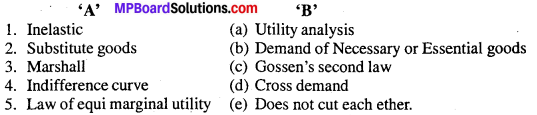1. (b)
2. (d)
3. (a)
4. (e)
5. (c).

Question 5.
Answer the following in one word / sentence:

1. Which curve is convex to the origin?
2. After which stage marginal utility and Total utility starts decreasing?
3. The point where a consumer derives maximum satisfaction is known as?
4. The elasticity of demand of luxurious goods is.
5. What is the demand for means of productions?

1. Indifference curve
2. At zero point
3. Consumer’s equilibrium
4. Highly elastic
5. Derived demand.

Consumer Behavior and Demand Very Short Answer Type Questions

Question 1.
What is the law of demand?
The law of demand states that other things remaining the same, quantity demanded increases with the fall in price and quantity demanded decreases with rise in price.

Question 2.
What are complementary goods?
Those goods which are jointly required to satisfy a particular want are known as complementary goods, e.g., bread and butter, car and petrol, pen and ink. These are also known as joint demand.

Question 3.
What is elasticity of demand?
When we measure the proportionate change in the quantity demanded of a commodity due to change in its price, is known as elasticity of demand.

Question 4.
What do you mean by Relatively elastic demand?
Demand is said to be relatively elastic when percentage change in demand is greater than percentage change in price.Question 5.
What is perfectly inelastic demand?
The change of price does not affect the demand of certain commodities. The demand for these commodities remain almost constant. The demand of these commodities are known as perfectly inelastic demand (Ep = 0).

Question 6.
Write the differences between the demand and want
Differences between the Demand and Want:
Demand:

1. Demand is created by want.
2. Demand goes on changing.

Want:

1. Desire gives rise to want.
2. Wants are more or less permanent.

Question 7.
What is cross elasticity of demand?
Cross elasticity of Demand :
Cross elasticity of demand refers to a change in demand for a goods as a result of change in the price of another goods. Following formula for the cross elasticity of demand :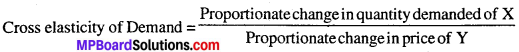Cross elasticity of demand arises in case of interrelated goods such as substitutes and complementary goods.

Question 8.
What are substitute goods?
Commodities which can be used in place of other goods are known as substitute goods. Fall in price of one commodity leads to rise in demand for other commodity.
Example : Tea and coffee, complain and bournvita.

Question 9.
What do you mean by consumer’s equilibrium?
It refers to a situation under which a consumer spends his given income on purchase of a commodity in such a way which gives him maximum satisfaction and there is no tendency to change.

Question 10.
What is the effect of increase or decrease in the level of income on elasticity of demand?
With the increase in income demand increases and with the decrease in income demand falls.

Question 11.
What do you mean by the budget set of a consumer?
The budget set is the collection of all bundles of goods that a consumer can buy with his income at a prevailing market price.

Question 12.
What is budget line?
The budget line represents all bundles which cost the consumers his entire income. The budget line is negatively sloping. If the consumer wants to consume an extra unit of goods 1, he will have to give up some amount of goods 2.

Question 13.
What do you mean by indifference curve?
An indifference curve is the curve which represents all the combinations of two goods which gives the same level of satisfaction to a consumer.

Question 14.
What do you mean by market demand curve?
Market demand curve represents the total of quantities of a commodity demanded by all the consumers in the market at different prices.

Question 15.
What do you mean by zero elasticity of demand or perfectly inelastic demand?
A perfectly inelastic demand is one in which a change in a price causes no change in the quantity demanded.

Question 16.
What do you mean by market demand?
Market demand means the total quantity of a good that all its buyers are willing to purchase at different prices over a given period of time.Question 17.
What do you mean by Giffen goods?
or
What do you mean by inferior goods?
Giffen goods may be defined as those goods whose price effect is positive and income effect is negative. In it the demand falls with the fall in price and rises with an increase in price.

Question 18.
What do you mean by price effect?
When the price of a commodity falls the real income of the consumer goes up and thus demand of the commodity increases. This effect is called the price effect.

Question 19.
Write the assumptions of the demand curve.
Following are the assumptions of demand curve:

1. There should not be any change in income and interest of consumer.
2. It is assumed that there is small and continuous change in price and demand relationship.
3. Goods can be divided into small units.
4. Perfect completion is found in the market.

Question 20.
What do you mean by normal goods?
Normal goods:
Normal goods are those goods for which the demand increases with the increases in income of the consumer and decreases with fall in income.
For example :
Demand for such goods like car, refrigerators, televisions, etc. increases with the increase in income and their demand decreases with the decrease in income.

Question 21.
Explain the meaning of complementary goods. Explain with example.
Complementary goods are those goods which are complementary to one another. In other words, they are used jointly or consumed together. For example: Ink and Pen, Car and Petrol. An increase or decrease in price of petrol will affect the use of car. If the price for petrol decreases, the demand for car will increase along with demand for car. On the other hand, if the prices of petrol increase the demand for petrol will decreases along with the demand for car.Question 22.
Consider the demand curve Dp = 10 – 3x. What is the elasticity at price
$$\frac{5}{3}$$?
Solution:
Given ; q = a – bp
q = 10 – 3p
a = 10, b = 3, p =$$\frac{5}{3}$$
eD=-$$\frac{bp}{a-bp}$$
=(3 x $$\frac{5}{3}$$) ÷ (10 – 3 x $$\frac{5}{3}$$)
= – (5) ÷ (10 – 5) = – 5 – 5 – 5 = -1
Price elasticity is (Ed) = -1
Price elasticity = -1.

Question 23.
Write the difference between Law of Demand and Elasticity of Demand.
Differences between Law of Demand and Elasticity of Demand:
Law of Demand:

1. The Law of demand is only a qualitative statement.
2. It indicates direction of change in demand in response to the change in its price.
3. It does not specify the quantity of change in demand in response to the change in price.

Elasticity of Demand:

1. It is a quantitative statement.
2. It indicates quantitative change in the demand for a commodity in response to a change in its price.
3. Elasticity of demand is a measure of rate of change in demand in response price. to a change in the price of a commodity.

Question 24.
What do you mean by income demand?
The income demand refers to various quantities of goods and services which would be purchased by the consumer at various levels of income. We assume that the price of the commodity or service as well as the price related goods the taste and desire of the consumer do not change. The income demand shows relationship between the income and quantities demanded. If the income increases the demand for a particular good will be increased and vice versa.

Question 25.
What do you mean by income elasticity of demand? Give one definition.
The income and demand of goods has direct relation. The income elasticity of demand means the change .in demand which occurs as a result of change in income. Here, price remains constant.

Definition of Watson:
“Income elasticity of demand in the ratio between percentage of change in income and % of change in quantity demanded.”

Question 26.
Define price elasticity of demand.
It is a measure of degree of responsiveness of demand for a commodity to change in its price or it is the ratio of percentage change in quantity demanded in response to a percentage change in price.

Consumer Behavior and Demand Short Answer Type Questions

Question 1.
What do you mean by utility? Give definition of it.

1. The power or capacity which satisfies human wants is called utility.
2. According to Prof. Waugh, “Utility is the capacity to satisfy human wants”.Question 2.
Explain the characteristics of utility. Give definition of it.
1. Utility is a psychological word :
Utility is a formless thing which is concerned with human sentiments. It can only be experienced because it has no form. Therefore, utility is supposed to be a psychological word and that the utility of one commodity may be different for different persons. It is an abstract noun. .

2. Utility is an individual characteristic:
The second characteristic of utility is that it is personal. The same utility is found to be different for different individuals. The utility of a commodity for one individual depends on his desire, taste, fashion, habit, environment and such other circumstances.

3. Utility depends on intensity of want:
The utility of a commodity depends on the intensity of its want. A person will obtain utility of a commodity depending upon the intensity of the utility of the commodity to the individual. This is the reason why a man gets maximum utility from food when he is very hungry. But the utility becomes zero when his hunger is satisfied.

Question 3.
Explain the types of utility.
There are three types of utility:

1. Total utility
2. Marginal utility
3. Average utility.

1. Total utility:
When a consumer uses more than one unit of a commodity, the sum total of the utility derived from all the units is called total utility. In other words, the utility derived from all the units of a commodity is called total utility.

2. Marginal utility:
The utility obtained from the last unit of a commodity is called marginal utility.“The marginal utility is the utility derived from the last unit of commodity consumed”.

3. Average utility:
When total utility of an article is divided with the total units of an article. The sum received is called average utility.

Question 4.
What are the factors which affect the marginal utility?
Following are the factors which affect the marginal utility:

1. Quantity of goods:
When the supply of a commodity is in surplus its marginal
utility declines on the other hand if there is scarcity of goods, the marginal utility will increase. .
2. Substitute goods:
If there are more substitutes available of a commodity, the marginal utility of that commodity declines and vice-versa.
3. Income of a person:
With the in the income of a person, the marginal utility of a commodity increases, while on the other hand it may decrease.

Question 5.
Write the assumptions of consumer’s equilibrium.
Following are the assumptions of the consumer’s equilibrium:

1. Maximum satisfaction :
The first assumption on of the consumer equilibrium is that consumer is a rational human being and by purchasing two goods, he can achieve maxi-mum satisfaction.
2. No change in taste and habit of the consumer during analysis:
It is assumed that during the time of analysis of consumer equilibrium, there is no changes in taste, habit and liking of the consumer.
3. Perfect competition:
There is perfect competition in the market from where he purchases his goods.
4. Consumer has an indifference map:
Consumer has an indifference map showing his scale of preference for various combinations of the two goods (Apples and Mangoes). This scale of preference remains the same through out the analysis.
5. No change in the price of the commodity:
Prices of the goods in the market are given and they are constant during consumer’s equilibrium. Each goods is homogeneous and divisible
6. 6. Constant amount of money:
Consumer is given a constant amount of money to spend on the goods and if he does not spend on one good he must spend it on other.Question 6.
Write three importance of law of diminishing marginal utility.
Following three importance of the law of diminishing marginal utility is of great use to man in almost every walk of life:

1. Basis of production:
The law of diminishing marginal utility helps the producers to produce their articles and goods they know that,the consumer gets the diminishing utility by consuming successive units of a commodity. So, various types of articles are produced by him instead of same articles.

2. Basis of consumer’s surplus:
The law helps us to study consumer’s behavior. We know that by each successive unit gives less and less utility to the consumer, though the price of the commodity is the same. The consumer thus stops consuming the commodity at the points of satisfaction.

3. Law of demand:
The law of diminishing marginal utility is the basis of the ‘Law of demand. It is this law which explains to us why the demand curve slopes downwards. The diminishing tendency is utility with increase in supply shows the points cut by the price and amount demanded in.

Question 7.
Write defects of law of diminishing marginal utility.
The law of diminishing marginal utility is not free from the defects.

1. Utility cannot be measured cardinally:
According to Marshall, utility analysis is based on the hypothesis that utility is cardinally measured in utils or units and that utility can be added and subtracted.

2. Marginal utility of money is not constant:
The utility analysis assumes the marginal utility of money to be constant. Critics point out that marginal utility of money never remain constant. So it may affect consumer’s preferences.

3. The law depends on the supply of the substitutes and complements:
The law does not depend upon the supply of the commodity a consumer consumes but also on its substitutes and complements. If the green coconuts are available in plenty the utility of sarabat will decrease rapidly.

4. Rationality:
The theory assumes that the consumer is rational. However, various factors such as advertisement and ignorance can influence the consumer’s decision.

5. In-applicability in case of indivisible goods:
The application of this law to an indivisible bulky commodity seems to be absurd because no one would normally but at a time more than one unit of goods like TV set, Scooter, House etc.

Question 8.
Write the essential elements of demand.
Following are the essential elements of demand:

1. Desire for goods: For demand desire of any good is essential. If a person does not have desire for anything, then it cannot be turned into demand.
2. Sufficient means or Wealth to purchase:
A person should have sufficient wealth and means to get the goods, otherwise he/she cannot fulfill his wants.
3. Eagerness to spend:
A person should be willing to use means or wealth to fulfill his demand. Otherwise it will remain only desire not the demand.
4. Fixed price:
Demand is always expressed at a fixed price. If it is not expressed in terms of price, we cannot call it demand.
5. Fixed Time:
Demand is always expressed at a particular time. In a specific time only quantity of good is demanded.

Question 9.
Distinguish between Marginal Utility and Total Utility.
Differences between Marginal Utility and Total Utility: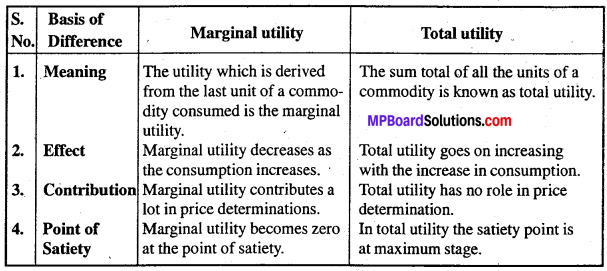Question 10.
Explain, why the budget line is downward sloping?
A budget line is the line showing different possible combinations of two goods i.e., goods x and goods y, which a consumer can buy, given his budget and the prices of two goods. Now, when the consumer can purchase two goods only with the given income and the prices, it is but obvious that the consumer can buy more of goods x only when he buys less of goods y. So, the budget line will be downward sloping indicating an inverse relationship between the consumption of two goods.

Question 11.
Write the exceptions of law of demand.
Following are the exceptions of the law of demand:

1. Articles of Distinction:
This exception was first of all discussed by Veblen. According to him, articles of distinction have more demand only if their prices are sufficiently high. Diamond, jewelry, costly carpets etc. have more demand because their prices are abnormally high. It is so because distinction is bestowed on diamond, jewelry, etc. by the society because of their being costly. Accordingly, their demand is also high. If their prices falls, they will no longer be considered as articles of distinction and so their demand will decrease.

2. Giffen Goods:
In other words, Giffen goods are those inferior goods in the case of which income effect is negative and stronger than the substitution effect of a change in price. As a result, when price of such commodities falls, their demand also shrinks. Both in case of inferior good and Giffen goods income effect is negative. But in case of Giffen goods, negative income effect is always stronger than the substitution effect.

While in case of inferior goods, it may or may not be so. Law of demand fails only if negative income effect is stronger than the substitution effect. So, that while law of demand may or may not fail in case of inferior goods, it must always fail in case of Giffen goods. Therefore, at higher price the quantity demand of diamonds by rich consumers may increase.

3. Expectation regarding future prices:
If price of a commodity is rising today and it is likely tor rise more in the future, people will buy more even at the existing higher price and store it up. They will do this order to avoid the pinch of higher price in future. Similarly, when the consumers anticipate a large fall in the price of a commodity in future, they will postpone their purchase even when price falls today so as to purchase this commodity at a still lower price in future.

4. Emergencies:
Law of demand may not hold good emergencies like war, famines, etc. At such times consumers behave in an abnormal way. If they expect shortage of goods, they would buy and hoard goods even at high prices during such periods. On the other hand, during depression they will buy less even at low prices.

Question 12.
A consumer wants to consume two goods the prices of two goods are Rs. 4 and Rs. 5, respectively. The consumer incomes is Rs. 20. Find out the following:

1. Write down the equation of the budget line.
2. How much of goods 1 can the consumer consume if he/she spends entire income on that goods?
3. How much of goods 2 Can a consumer consume if he/she spends her entire income on that goods?
4. What is the sole of the budget line?

Equation of budget line is:
1. Px Qx + Py Qy < Y, 4 Qy ≤ 20.

2. If he/she spends entire on goods 1 then his consumption on 1 will be,
= Total income of consumer =$$\frac{Rs.20}{Rs.4}$$
= Price of one goods.
= 5 units.

3. If the consumer consumes his full income on goods 2 then he will consume goods 2 in this quantity.
= Total incomes of consumer =$$\frac{Rs.20}{Rs.4}$$
=4 units.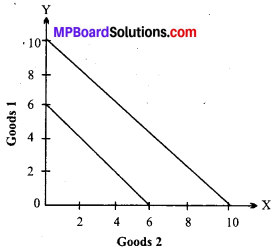Question 13.
How does the budget line change if the consumer’s income increases to ₹40 but the prices remain unchanged? (NCERT)
An increase in consumer’s income implies that the consumer can purchase increased quantities of both the commodities at the prevailing market price. As a result the consumer will face a new budget line. The new budget line will shift-rightwards parallel to the original budget line. It can be explained with the following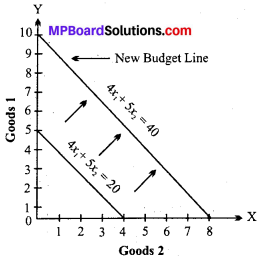In the above figure if the income of consumers is increased from ₹ 20 to ₹ 40 the budget line shift/upward) right side. Now the consumer can consume more quantity of goods than before because there is no change in price of goods. The new budget line is parallel to the original budget line.

Question 14.
How does the budget line change if the price of good 2 decreases by a rupee but the price of good 1 and the consumer’s income remain unchanged?
If the price of goods 2 decreases by ₹1 and there is no change in income and price the budget line will change because the consumer can buy more of goods 2. It is clear form the following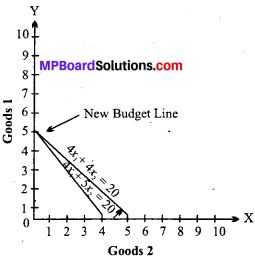Question 15.
Suppose a consumer can afford to buy 6 units of goods 1 and 8 units of goods 2 if he spends his entire income. The prices of two goods are ₹6 and ₹8 respectively. How much in the consumer’s income?
Price of good 1= ₹6.
Quantity of good = ₹6.
Price of good 2 = ₹8.
Quantity of good 2 = ₹8.
Budget set;
or C1 X1 + C1 X2 = I
or 6 x 6 + 8 x 8 = I
or 36 + 64 = 1
100 = 1
So, income of consumer.

Question 16.
Consider the demand curve for a good. At price ₹ 4 the demand for the good is ₹ 25. Suppose the price of the good increases to₹ 5 and as a result the command for the falls to 20 unit Find out the price elasticity.
Solution: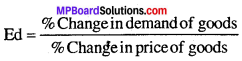=$$\frac { \frac { { q }_{ 1 }-{ q }_{ 0 } }{ { q }_{ 0 } } }{ \frac { p_{ 1 }-{ p }_{ 0 } }{ { p }_{ 0 } } }$$
According to question, q0 = 25, p0 = 4, q0 = 20, p1 = 5
$${ e }_{ D }=\frac { \frac { 20-25 }{ 25 } }{ \frac { 5-4 }{ 4 } } =\frac { \frac { -5 }{ 25 } }{ \frac { 1 }{ 4 } }$$
$${ e }_{ D }=\frac { -5 }{ 25 } x\frac { 4 }{ 1 } =\frac { -20 }{ 25 } =-0.8$$

Question 17.
Write the assumptions of the law of marginal utility.

1. Units of the commodity must be similar:
Each successive unit of the commodity must be similar in quantity and quality.
2. Consumption should be continuous:
The law will operate only when the consumption of unit is continuous without interval.
3. Interest nature of the consumer should not change:
If in the period of consumer interest and nature of the consumer changes then the law will not be applicable.
4. Income should not change:
The income of the consumer should not be changed. As soon as income changes man’s need changes.

Question 18.
Suppose the price elasticity of demand for a good is 0.2. If there is a 5% increase in the price of the good, by what percentage will the demand for the good go down?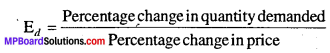Substituting the value, we get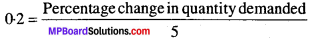or, percentage change in quantity demanded
= 0.2 x 5 = 1
Demand of good will be reduced by 1 %.

Consumer Behavior and Demand Long Answer Type Questions

Question 1.
Write any six causes of the implication of the law of demand.
Or
Why demand curve slopes downwards to the right?
It is a general law that the demand curve slopes downwards to the right. It shows the inverse relationship between the price and demand i.e., when price rises demand falls and vice – versa. This is why, it is also known as negative slope of the demand curve.

Following are the causes responsible for the downward sloping of the demand curve:

1. Law of diminishing marginal utility:
According to this law, consumption of a commodity increases, the utility from each successive unit goes on diminishing. Accordingly for every additional unit to be purchased, the consumer is willing to pay less price.

2. Income effect change:
Change in own price of a commodity causes a change in real income of the consumer, with a fall in price, real income increases. Accordingly, demand for the commodity expands.

3. Substitution effect:
It refers to substitution of one commodity for the other when it becomes relatively cheaper due in relative prices.

4. Size of consumer group:
When price of a commodity falls, it attracts new buyers who can afford to buy it, hence, quantity demanded rises.

5. Different uses:
Many goods have alternative uses e.g., Milk is used for making Curd, cheese and butter. If price of milk reduces, it will be put into different uses. Accordingly, demand for milk expands.

6. Tendency to satisfy the unsatisfied wants:
Each person has some unsatisfied wants. When the price of the goods falls, he wants to satisfy his unsatisfied wants which leads him to increase its demand. Because of this tendency of human beings, the demand curve slopes downwards to the right.

Question 2.
Write any five exceptions to the law of demand.
The law of demand i.e., more demand of the commodity at lower price and lesser demand at higher price does not apply in every case and situation. The exceptions to the law of demand are as follows :
1. Necessaries of life:
Certain commodities represent necessaries of life and so the consumer is forced to buy them even at high price. As such, the demand curve may go upward or the law of demand may not operate.

2. Social status symbol:
There are certain goods which constitute social status symbol and the consumer has to buy them for maintaining his prestige in the society. He is, therefore, compelled to purchase such goods inspire of an increase in their prices. The law of demand will not operate as such.

3. Precious stores:
The law of demand does not operate in the case of precious stones like diamond etc. People do purchase them inspire of an increase in their price in order to distinguish their recognition.

4. Ignorance and psychology of consumer:
If the consumer is not aware of the competitive price of a commodity prevailing in the market, he may buy it in more quantity at higher price. It may be a psychological phenomenon that high price represents high quality. The law of demand may not operate as such.

5. Change in fashion, habits, interest and preference:
The law of demand does not operate when there is a change in consumer’s taste, habit, interest, fashion and preference. A commodity of new fashion will be purchased more even if the price is increased and so also is the case of change in habit, interest, taste and preference.Question 3.
Write any five factors affecting elasticity of demand.
The elasticity of demand is affected by the following factors:
1. Nature of commodities:
Demand for necessity is inelastic whereas the demand for luxuries is elastic. Here too, we have to note the possibility that substitution plays an important role. Demand for necessities like bread and potatoes is inelastic because there are no close substitutes for them within the same price range and not merely because they are necessaries”. Hanson further sates that “The demand for some expensive luxury goods may be very inelastic not because they are luxuries but rather because they lack close substitutes.”

2. Consumer’s income:
Elasticity is closely related to person’s income. The demand of rich for all commodities may be quite unaffected by any changes of price. But this is not the case with the majority of the people as they have limited income and have to make a choice between this or that commodity. They cannot purchase all commodities.

3. Proportion of income spent on commodity:
The demand for a commodity is quite elastic over which consumer’s spend a large part of their income. It is so because even a small rise in price is likely to reduce substantially their ability to buy this item and so result in sharp percentage declines in the quantity demanded.

4. The possibility of substitution:
The most important influence on elasticity of demand is the degree of closeness of the substitute for the goods. The closer the substitute, the higher the elasticity of demand.

5. Possibility of postponement of the use of commodity:
If the consumption of a commodity can be postponed for further, the demand for such commodity will be elastic as the consumer does not feel its urgency. On the other hand if the consumption of a commodity cannot be postponed for future or which is very urgent, the demand for it will be inelastic.

Question 4.
Explain the factors affecting demand.
Following are the factors affecting demand:

1. Income:
When consumer’s income increases, he or she usually buys more goods which increases the demand.
2. Price of substitute goods:
When the price of substitute goods increases, a consumer normally gives up at least some of its consumption and as a result the demand in-creases. (e.g., pineapple).
3. Prices of complementary goods:
When the price of complementary good in-creases, a consumer normally gives up some of its consumption as a result demand decreases (e.g. sugar).
4. Number of consumer:
When the number of consumers increases there are more people who buy the goods and as a result demand increases.
5. Consumer’s taste:
When a consumer likes the goods more he or she buys it more and the demand increases.

Question 5.
Explain the types of price elasticity of demand.
Or
Explain the degree of price elasticity of demand.
Following are the types of price elasticity of demand:

1. Perfectly elastic demand:
Let us take one extreme case of elasticity of demand, when it is infinite or perfect elasticity of demand is infinity when even a negligible fall in the price of commodity leads to an infinite extension in the demand for it. Even when the price remains the same, the demand goes on changing.

2. Perfectly inelastic demand:
It means however great the rise or fall in the price of the commodity, its demand remains absolutely unchanged. In
other words, the elasticity of demand is zero. No amount of change in price induces a change in demand.  In other words, the change in price does not at all affect the quantity demanded. It is a case of perfectly inelastic demand.

3. Unitary elastic demand:
Demand for a commodity will be said to be unitary elastic, if the percentage change in the quantity demanded equals to the percentage change in the price. In other words, when the demand changes in the same proportion as in the price of the commodity, the elasticity of demand is equal to one.

4. Inelastic demand or Less than unit elastic demand:
When a considerable change in price does not lead to much change in the demand, the demand is said to be less elastic or inelastic. Here, the elasticity of demand is said to be less than unity. The slope of demand curve is more inclined towards Y axis.

5. Elastic demand or More than unit elastic demand :
When a small change in price leads to a greater change in demand, the demand is said to be elastic or more elastic. Here, the elasticity of demand is said to be greater than unity. In this case, proportionate change in demand will be more than proportionate change in price.Question 6.
Explain the law of demand with the help of table and graph.
The law of demand explains the relationship between price of a commodity and its quantity demanded in the market. It says that there is inverse relationship between price and the quantity demanded. The law states that other things remaining same, the consumer will demand lesser quantity of goods at higher price and more quantity of goods at lower price. Thus, consumer’s equilibrium is the basis of law of demand.
Example: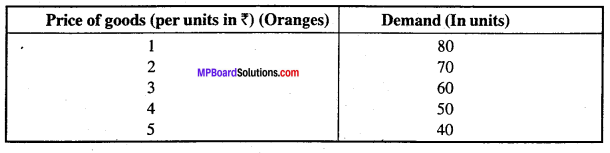The above table show that when the price of oranges is ₹ 1 per unit 80 units are demanded. If the price increases to ₹ 5 the quantity demand decreases it is 40 units.

This can be further explained with the help of figure:
From the figure it is clear that DD demand curve is a downward slopping curve. Which indicates that when the price of a good increases demand falls and when price reduces there is increase in demand. This shows inverse relation between price and demand. Demand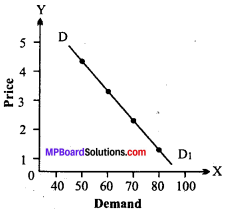Question 7.
Explain the law of diminishing marginal utility with example.
Definition of Law of Diminishing Marginal Utility:

1. Prof. Alfred Marshall:
“The additional benefit which a person derives from a given increase in his stock of a thing diminishes with every increase in the stock that he already has.”

The law of diminishing marginal utility has great importance in the study of economics. This law was firstly introduced by the French economist Herman Henrick Gosen. So, it is known as ‘Gosen’s first-law, Afterwards Prof. Marshall presented it scientifically in a systematic manner. This law explains the relation between the units of a commodity and it marginal utility.

The law is the most logical exposition of a consumer behavior. As we go on consuming more of anything in succession, the satisfaction derived by its successive units goes on decreasing. It is a matter of common observation that the more we have the commodity, the less urgently we want its subsequent units.

Explanation: We may take an example to illustrate this law. We are very hungry and we want food. We start eating bread. The first bread, that we eat is of utmost importance to us. The utility of this bread is say 40. Then we eat the second bread, but the utility of this bread is not as great as that of the first. Its utility is say 30, because our hunger has been slightly satisfied. The next bread that we eat have lesser and lesser of utility. The utility of the third and fourth bread is say 20 and 10 respectively.

Suppose after eating 5 units of bread his hunger is satisfied. Now we won’t like to eat the sixth bread. There will be no intensity to eat the sixth bread, therefore no utility of the sixth bread will be there. Still if we eat the sixth bread, we may get displeasure or dissatisfaction. This is the negative utility.Here we get zero utility. The seventh bread will give him negative utility because he has spend money on it without getting any satisfaction. Because of the consumption of the additional unit he might feel uneasy.

We may summarize it in this manner :

1. As long as we have intensity for a thing, there will be utility for that.
2. With the consumption of each unit of any commodity, the intensity for that commodity will be lesser and lesser, hence its utility will also diminish.
3.  If there is no intensity for a commodity, there will be no utility.
4.  In spite of no intensity if we keep on consuming a thing, there will be negative
utility.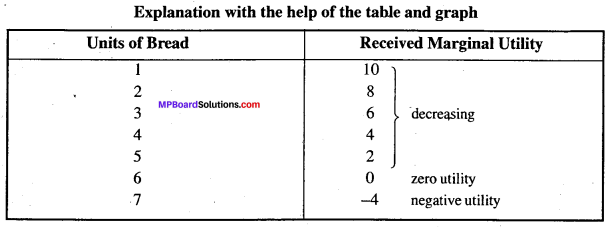In the above example, we see that after consuming number of 5 breads, our hunger is satisfied, therefore the utility of bread will be up to 5 breads only. This utility is in the decreasing order because with the consumption of every bread his hunger also decreases. At the consumption of the sixth bread, the utility received is zero because the hunger has been fully satisfied. There is no need of the seventh bread, but still if he eats, there will be negative utility.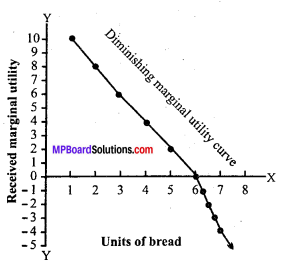Question 8.
In economics Giffen goods is a good that is in greater demand as its price increases and falls when the price decreases. A Giffen goods is typically an inferior product that does not have easily available substitutes. There is a positive relationship between the price and the quantity demanded.

This situation is also known as Giffen paradox. The demand for inferior goods such as coarse grain, coarse cloth, inferior meat etc., will not increase even with the fall of their price, because consumers of there commodities will start consuming more of a superior commodity. As a result, the demand for Giffen commodity will fall.Question 9.
Explain the concept of consumer’s equilibrium with the help of suitable example.
Consumers equilibrium refers to a situation, in which a consumer derives maximum satisfaction, with no intention to change it and subject to given prices and given income. The point of maximum satisfaction is achieved. The consumer equilibrium under indifference curve must meet the following conditions:

The three conditions must be satisfied for a consumer to attain equilibrium:

1. The price line should be tangent to an indifference curve or MRS of one commodity for another should be equal to their relative prices.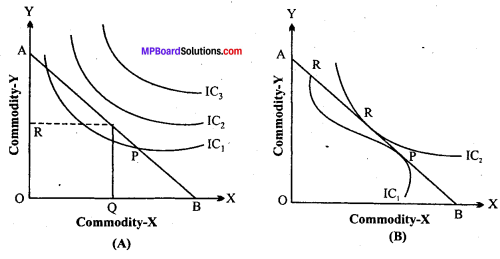AB is the price line and IC1, IC2 and IC3 are the indifference curves. This curve shows the combination of goods X and Y. IC3 and IC2 indifference curve which lie above and below the price line. In other words a rational consumer with limited income cannot do the expenditure and curve IC1 shows less satisfaction, but IC2 curve touches the price line at ‘P’ which is a point at which consumer maximizes his satisfaction.

2. In the given figure, slope of price line and indifferent curve is same or equal at point ‘P.’

3. At the point of equilibrium an indifference curve must be convex to the origin. Thus, the point of consumer equilibrium or maximum satisfaction may be defined by the condition that the marginal rate of substitution between any pair of two commodity will be equal to the ratio of their prices, or the marginal rate of substitution of money for any commodity is equal to the price of the commodity.

Question 10.
What is indifference curve? Write its features.
An indifference curve is the curve, which represents all those combinations of two commodity which give same level of satisfaction.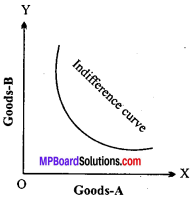Main features are :

1. The indifference curve must slopes downwards to the right.
2. Higher indifference curve represents higher level of satisfaction.
3. Indifference curves are convex to the origin.
4. Indifference curve cannot intersect each other.
5. Indifference curve do not touch the horizontal or vertical axis.
6. The consumer acts rationally so as to maximize satisfaction.
7. There are two goods X and Y.
8. The consumer possesses complete information about the prices of the goods in the market.Question 11.
What is indifference map?
A set of indifference curves is called indifference map. An indifference map depicts complete figure of consumer’s tastes and preference. The indifference map shows the preference of the consumer, he definitely prefer’s the combination lying on the higher indifference curve to the combination lying on a lower indifference curve because a higher indifference curve represents a higher level of satisfaction.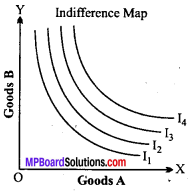Question 12.
What do you mean by extension of demand and contraction of demand?
1. Extension of demand :
When the quantity demanded of a commodity rises due to fall in its price, other things remaining the same, it is called ‘rise in quantity demanded or extension of demand. For example, as shown in when the price of apples falls ₹ 30 per Kg to ₹ 25 per Kg. A consumers purchase of apples rises from 1 Kg to 2 Kg per week. This is extension of demand.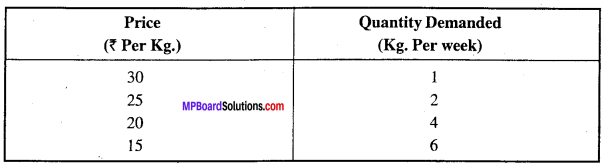2. Contraction of demand:
Contraction of demand or fall in quantity demanded refers to a fall in quantity demanded of a commodity as a result of rise in its price, other things remaining the same.” When the price of apples rises from ₹15 a Kg. to ₹ 20 a Kg. a consumer buys less apples, 4 Kg. instead of 6 Kg. In this case, there is contraction of demand or decrease in quantity demanded.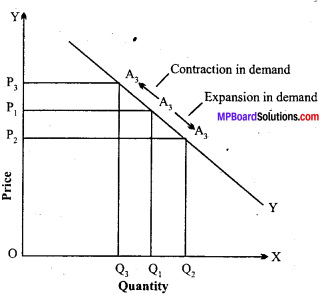Any point on a demand curve represents a particular quantity being bought at a specified price. Different points on a demand curve represent quantities demanded at different prices. Therefore, a change in quantity demanded is indicated by a movement along a particular demand curve. A movement down a demand curve is called a “rise in quantity demanded” or “extension of demand.”

On the other hand, a movement up the demand curve is called a‘ fall in quantity demanded of ‘contraction of demand.’ Thus, a change in the quantity demanded as a result of change in price of a commodity alone does not involve the drawing of a new demand curve, but is represented by the movement up or down on a given demand curve. This is illustrated in.

It will be seen in fig. that when the price is OP1 quantity demanded is OQ1. Now, if the price of the commodity falls to OP2, the quantity demanded rises to QO2. This movement from A1 to A2 in the downward direction (as indicated by an arrow) on the given demand curve DD is the extension of demand.

On the other hand, if the price of the commodity rises from OP1 to OP3, the quantity demanded of the good falls to OQ3. This movement from A1 to A3 in the upward direction (as indicated by the arrow) on the given demand curve is the contraction of demand.

Extension in demand results from
a fall in the price, other things remaining the same.

Contraction in Demand results from
a rise in the price, other things remaining the same.